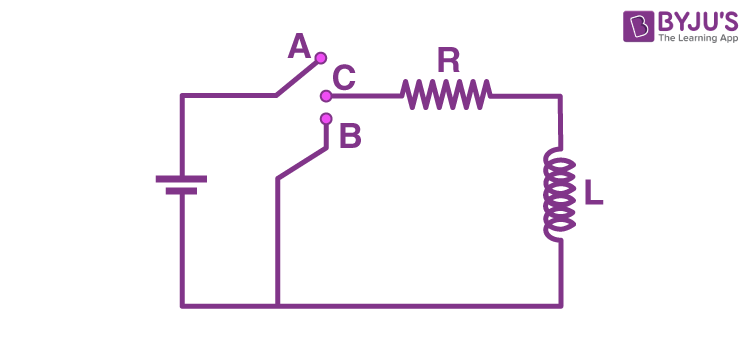# In the circuit shown here, the point 'C' is kept connected to point 'A' till the current flowing through the circuit becomes constant. Afterward, suddenly, point 'C' is disconnected from point 'A' and connected to point 'B' at time t=0. Ratio of the voltage across resistance and the inductor at t =L/R will be equal to:a) 0

b) 1

c) -1

d) infinity

Solution:’

Let the emf of the battery be E.From the question, the point C of the circuit is connected to the point A. So the circuit will look like

So the inductor is connected to the DC source. When a DC source is applied across an inductor, it behaves as a short circuit after the steady state is reached.

Current in the circuit at this instant is given by

I = E/R

Current through the LR circuit is given by

i(t) = i0exp(-t/τ) ——(1)

As the current cannot suddenly change through an inductor, so the current at the time instant t=0 will be equal to the current through the inductor before closing the circuit, that is,

i= I = E/R ———-(2)

i(t) = (E/R)exp(-t/τ) ——-(3)

We know that the time constant of an LR circuit is given by

τ = L/R ———-(4)

According to the question, the given instant of time is

t=L/R———(5)

From (4) and (5)

t=τ

Putting this in (3) we get

i(L/R)=(E/R)exp(−τ/τ)

⇒i(L/R)=E/Re ——–(6)

Now, we know from the Ohm’s law that the voltage across the resistance is

VR=IR

Putting (6) above, we get
VR=(E/Re)R

⇒VR=E/e……………….(7)

We also know that the voltage across an inductor is given by

VL=L(di(t)/dt)

From (3)

VL=Ld[(E/R)exp(−t/τ))]/dt

VL=(−EL/Rτ)exp(−t/τ)

Putting the value of the time constant from (4)

VL=(−ELR/RL)exp(−Rt/L)

⇒VL=−Eexp(−Rt/L)

At the time t=L/R we get the voltage across the inductor as

VL=−Eexp(−RL/LR)

⇒VL=−E/e………………….(8)

Dividing (7) by (8) we get

VL/VR=−1

Thus, the required ratio is equal to −1.(0)(0)